Courses

# Techniques MCQ

## 21 Questions MCQ Test Mock Test Series of IIT JAM Biotechnology | Techniques MCQ

Description
This mock test of Techniques MCQ for IIT JAM helps you for every IIT JAM entrance exam. This contains 21 Multiple Choice Questions for IIT JAM Techniques MCQ (mcq) to study with solutions a complete question bank. The solved questions answers in this Techniques MCQ quiz give you a good mix of easy questions and tough questions. IIT JAM students definitely take this Techniques MCQ exercise for a better result in the exam. You can find other Techniques MCQ extra questions, long questions & short questions for IIT JAM on EduRev as well by searching above.
QUESTION: 1

### A research scholar has a mixture of proteins with the following properties. Protein P → M.wt = 11 Kd; PI = 10 Protein Q →M.wt = 60 Kd: PI = 4 Protein R →M.wt = 26 Kd: PI = 8 Protein S→M.wt = 9 Kd; PI = 5 Predict the order of elution of these proteins when a mixture of the four proteins is separated by DEAE-cellulose chromatography at pH 7.

Solution: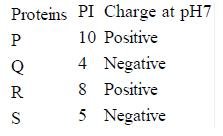As DEAE - cellulose is used that means fixed ion is positive charge in ion exchange chromatogram. So negative charge ions will be attracted and elute later.
So the P will elute first and then R and then S and finally Q.

QUESTION: 2

### The following gel pattern was obtained in an attempt to sequence a DNA molecule using the Sanger method. What is the sequence of the DNA molecule in question.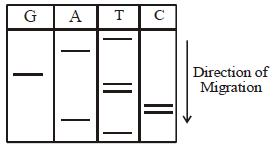Solution:

By observing the given autoradiogram the sequence will come of amplified DNA so it will come
5’TACCTTGAT3’. So the sequence of the given DNA would be 5’ATCAAGGTA3’.

QUESTION: 3

### Which of the following methods of DNA isolation is most suitable for isolation of genomic DNA from plants?

Solution:

Cetyltrimethylammonium bromide is a cationic detergent that enables quick precipitation of cell debris and other biomolecules and hence help in separation of DNA especially from plants.

QUESTION: 4

Which of the following is a non-parametric test?

Solution:

Non-parametric test refer to statistical tests which do not involve numeric values (ordinal) but other indices like genetic ratios, degree of pain, etc. Chi square test is one such example.

QUESTION: 5

DNA-protein interaction can be determined by

Solution:

DNA foot printing is use to identify DNA protein interaction. Bound protein in the DNA protect cleavage of DNA by nuclease. After banding pattern analysis it can be clearly observed.

QUESTION: 6

The purification of an antigen using the corresponding antibodies conjugated to agrose is an example of

Solution:

Affinity chromatography is the best method for protein purification. It is done by using specific affinity between the substrate to be isolated and molecule which can specific bind. So antigen can be purified by binding with antibody.

QUESTION: 7

There is mention the temperature of different steps of PCR, find out the correct match

Solution:

PCR is a technique which is use to amplify DNA invitro. It involves following steps.
(i) Denaturation at 94/95°C
(ii) Annealing at Tm± 4°
(iii) Extension at 72°C

QUESTION: 8

GST-tagged fusion protein will be purified by affinity chromatography by using which ligand?

Solution:

In affinity chromatography GST-tagged fusion protein can be purified by using Glutathione as ligand.

QUESTION: 9

Consider the following experimental distribution of a scientific data, obtained in one of the research lab, using flow cytometry method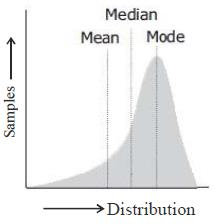One of the following inferences were drawn by the researcher is correct, mark the correct one.

Solution:

In case of unskewed or normal data, mean, median and mode coincide.

QUESTION: 10

Polyacrylamide gel electrophoresis is performed for

Solution:

Polyacrylamide gel electrophoresis is used to separate proteins and DNA less than 500 bp.

QUESTION: 11

Which of the following statements is not correct about gel electrophoresis?

Solution:

Electric field is dependent inversely on length of gel, it is related by the expression E = v/l, Where E is electric field, v is applied voltage and l is length of gel or distance between electrodes.

QUESTION: 12

Once, in a class of career endeavour academy having 225 students, weight of all the students was measured and data was represented in the form of mean±SEM as 70±3 If the all the data showed normal distribution, how many students are expected to have a weight exceeding 79 Kgs. would be approximately

Solution:

As per sigma rule a data which has normal distribution covers 68% of sample, hence weight in range of 70 ± 3 i.e. 67-73 would be covered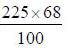i.e. 153 students. While 99.5% sample coverage would be observed when we consider mean ± 3σ, i.e. 70 ±9 would cover 99.5% sample, hence number of students above and below 79 ks would be 225-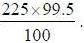Hence 1 student would be above 79 kg and 1 below 61 kg.

*Multiple options can be correct
QUESTION: 13

Which of the following methods can be used to determine the molecular weight of a given polypeptide.

Solution:

Gel filtration chromatography, SDS-PAGE, MALDI-TOF mass spectroscopy all three used in the molecular weight determination while ChIP assay is used for DNA protein interaction.

*Multiple options can be correct
QUESTION: 14

A scientist is working for the discovery of a biomarker for a particular disease. Find out the right options which he will used for this discovery.

Solution:

2-D gel electrophoresis is used for the discovery phase in proteomics approach, whatever the proteins have been identified by 2-D gel electrophoresis then these are validates by either ELISA or western blot.

*Multiple options can be correct
QUESTION: 15

There are methods which are used to study DNA-protein interaction

Solution:

Super shift assay, DNA foot printing EMSA and ChIP assay all are used for DNA, protein interaction.

*Multiple options can be correct
QUESTION: 16

Find the correct option from the following options

Solution:

Polymerase chain reaction (PCR) is used for amplification of DNA in laboratory condition and is very much used in forensic science laboratory for application purpose. Its first step is denaturation, then annealing and  finally extension.

*Multiple options can be correct
QUESTION: 17

Dideoxy DNA sequencing not exclusively depends on the following.

Solution:

Dideoxy sequencing method is also known as chain termination method because after addition of dideoxy nucleotide, the growing chain terminates because of the absence of OH group in dideoxy nucleotide.

*Answer can only contain numeric values
QUESTION: 18

One solution has absorbance of 0.50D, when path length of the cuvette is 1 cm. If this solution is diluted 10 times and 0.5 cm cuvette is used, the absorbance will be _______[Answer upto two decimal places]

Solution: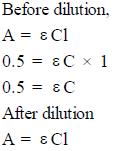...(i)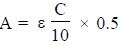...(ii)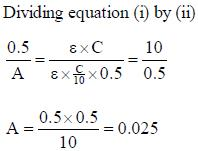*Answer can only contain numeric values
QUESTION: 19

Beginning with the 100 molecules of DNA templates, after 8 cycle of PCR, the number of amplicon will be ___________ [Answer in integer]

Solution:

Mf = m1 x 2n
= 100 x 2
= 100 x 256
= 25600

*Answer can only contain numeric values
QUESTION: 20

If the sequence of any DNA fragment is ATGCATGCATGCATGCATGC, its Tm (ºC) would be ___________ [Answer in integer]

Solution:

Tm = 4(G + C) + 2(A + T)
=4(10) + 2(10)
=40 + 20
=60°C

*Answer can only contain numeric values
QUESTION: 21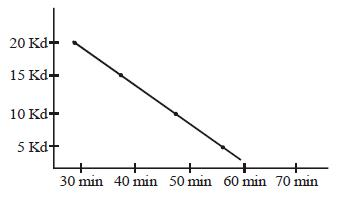This is the standard curve for gel filtration chromatography. If any unknown protein is eluting out from gel filtration chromatogram in 35 min, the approx. molecular weight of that protein will be ______  [Answer upto one decimal place]

Solution: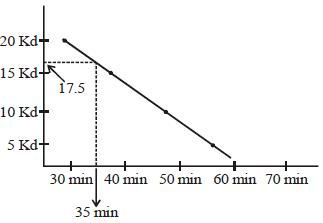Thats why17.5 Kd will be molecular weight.

Track your progress, build streaks, highlight & save important lessons and more!

### Similar Content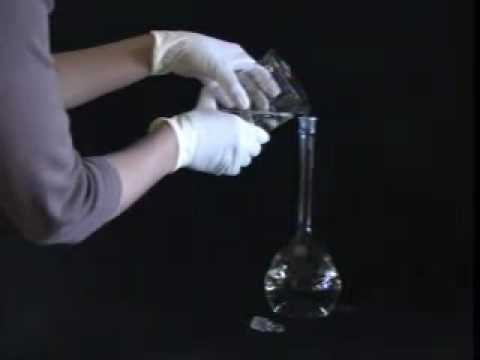### Related tests